# Temperature Converter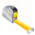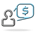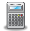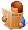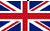Converter Currencies Calculator Articles

 Other units Area Energy Flowrate Force Length Mass Power Pressure Velocity Volume Temperature Time ______________ AcresAresBars Barrels per day Baryes BTUs BTUs per hour BTUs per minute BTUs per second Centimeters (cm) cm/sec Chains Cooking measures Crinals Cubic centimeters (cc) cc/sec cc-atmosphere Cubic decimeters Cubic feet Cubic feet per minute Cubic feet per second Cubic inches Cubic inches per minute Cubic inches per second Cubic meters Cubic meters per hour Cubic yards Cubic yards per minute Decimeters (dm) Drams DynesErgs per second Fluid drachmas Fluid ounces (fl.oz.) Feet (ft) Feet per hour Feet per minute Feet per second ft-lbf ft-lbf/hour ft-lbf/min ft-lbf/sec Furlongs Gallons Gallons per day Gallons per minute Gallons per second Gigawatts (GW) Gills Grains Grams (g) Gram-calories (g-cal) Hectares Hectometers Hundredweights (cwt) Inches (in) Inches H2O Inches Hg Inch-pound-forces (in-lbf) IST calories Joules (J) Joules per second (J/sec) Kilocalories (kcal) Kilograms (kg) kg-meters/sq. sec Kilogram-calories Kilogram-forces (kgf) Kgf/sq. cm Kgf/sq. meter Kilometers (km) Kilometers per hour (km/h) KiloPascal(kPa) Kilowatts (kW) Kilowatts per hour (kWh) Kips Knots Liters (l) Liters per minute Liters per second Long tons (imperial tons) MegaPascal(mPa) Megawatts (MW) Meters (m) Meters per minute Meters per second Metric horsepower Miles Miles per hour (mph) Miles per minute Milligrams (mg) Milliliters (ml) Millimeters (mm) Miner inches Newton (N) Ounces (oz) Ounce-force Pascal (Pa) Pints Pounds (lb) Pounds/sq. foot Pounds/sq. inch Poundals Poundals/sq. foot Poundals/sq. inch Pound-CHU Pound-force (lbf) Quarts Quarters Rods Shoe size Short tons/sq. footSlugs Square centimeters Square decimeters Square feet Square kilometers Square meters Square miles Square millimeters Square yards Stones Thermocalories TonsTons (refrigeration) Tonnes (metric tons) Torrs U.S. gallons U.S. horsepower per hour U.S. tons Watts Watts per hour Yards ________________ Ancient Greek: area Ancient Greek: currency Ancient Greek: length Ancient Greek: mass Ancient Greek: volume Ancient Roman: area Ancient Roman: length Ancient Roman: mass/coin Ancient Roman: volume Apothecary: imperial UK Apothecary: US/UK Apothecary: weightLeague units ________________ Calculator Contact Main website

 Select  Conversion Celsius  -->  Fahrenheit Celsius  -->  Kelvin Fahrenheit  -->  Celsius Fahrenheit  -->  Kelvin Kelvin  -->  Fahrenheit Kelvin  -->  Celsius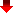Converting temperature units is important in many fields, including cooking, physics, engineering, and medicine. Understanding temperature in different units is also necessary when traveling between countries that use different systems of measurement. The two most commonly used temperature scales are Celsius and Fahrenheit. To convert from one to the other, a simple formula can be used. For example, to convert 100°C to Fahrenheit, the formula is (100°C x 9/5) + 32 = 212°F.

The Kelvin scale is used in scientific calculations and absolute zero, the lowest temperature that can be reached, is defined as 0 Kelvin or -273.15°C. The Rankine scale is used in thermodynamics and is based on the Fahrenheit scale, but with absolute zero defined as 0°R.

Proper conversion of temperature units is crucial in industries such as HVAC, where accurate temperature readings can impact safety, efficiency, and comfort. It is also important in the pharmaceutical industry where specific temperatures are required for the storage and transportation of sensitive products.

Overall, the ability to accurately convert temperature units is a valuable skill in many industries and everyday life.

MetricToImperial.com